# Conductor of a character

(diff) ← Older revision | Latest revision (diff) | Newer revision → (diff)

An integer associated to the character of a representation of the Galois group of a finite extension of a local field. Letbe a field that is complete with respect to a discrete valuation, with residue class fieldof characteristic. Let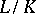be a Galois extension of degreewith Galois groupand suppose that the residue class field extension is separable. Ifis the character of some finite-dimensional complex representation of, its conductoris defined by the formula: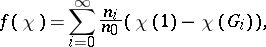where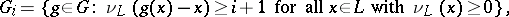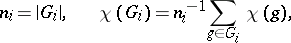whereis the corresponding valuation of. Ifdoes not divide, then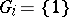for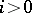and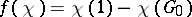. Ifis the character of a rational representation, then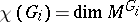. The conductor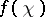is a non-negative integer.

How to Cite This Entry:
Conductor of a character. Encyclopedia of Mathematics. URL: http://encyclopediaofmath.org/index.php?title=Conductor_of_a_character&oldid=19015
This article was adapted from an original article by I.V. Dolgachev (originator), which appeared in Encyclopedia of Mathematics - ISBN 1402006098. See original article